#2

## 【pygame初體驗】學習用pygame來打造自己的遊戲

pygame是python語言中一個專門用來開發遊戲的模組，

# 二、在anaconda安裝pygame

【步驟一】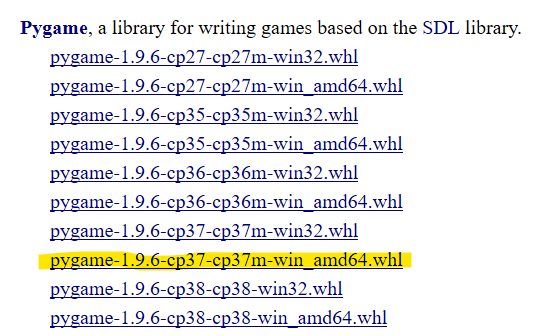【步驟二】

【舉例說明】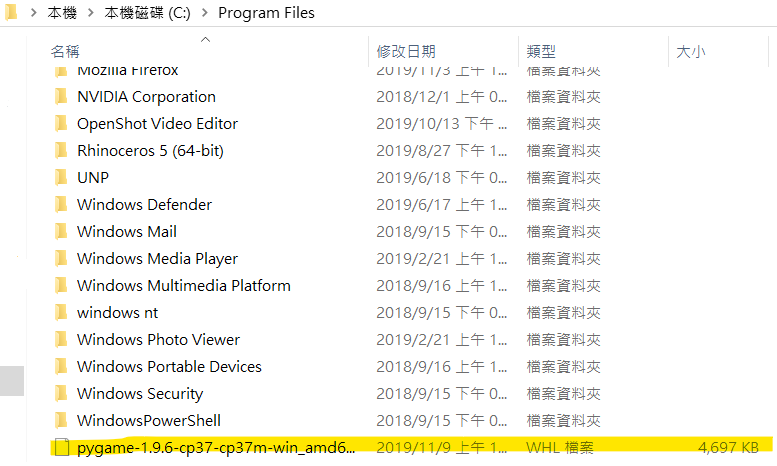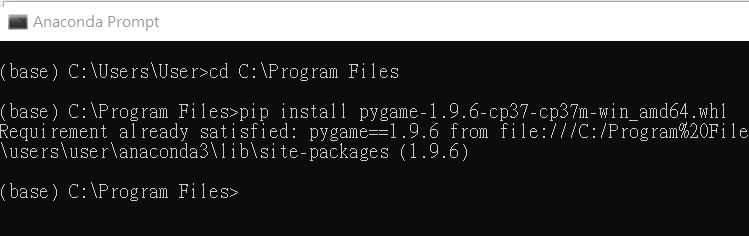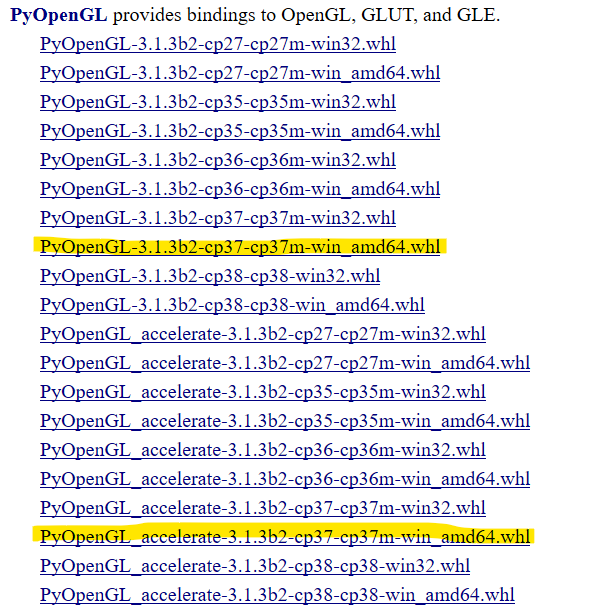# 三、基礎架構

「繪圖視窗」和「畫布」是分開的，

``````import pygame
pygame.init()  #pygame初始化

# 設定視窗
width, height = 640, 320
screen = pygame.display.set_mode((width, height))  #建立繪圖視窗
pygame.display.set_caption("基本架構")  #繪圖視窗標題

#建立畫布 (書上說通常不會直接在繪圖視窗畫圖)
background = pygame.Surface(screen.get_size())
background = background.convert() #為畫布建立副本，加快顯示速度
background.fill((255,255,255))  #畫布為白色(三個參數為RGB)
screen.blit(background, (0,0))  #在繪圖視窗繪製畫布

running = True
while running:  #無窮迴圈
for event in pygame.event.get():
if event.type == pygame.QUIT:  #使用者按右上角的關閉鈕
running = False
pygame.display.update()  #更新繪圖視窗

pygame.quit()  #關閉繪圖視窗
``````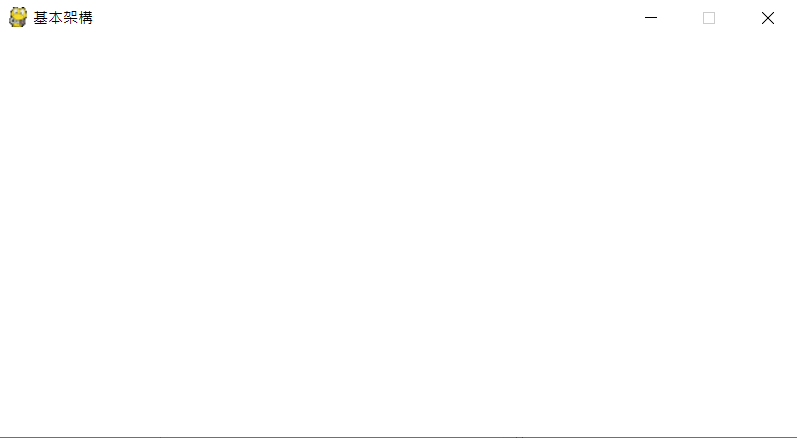``````# 錯誤示範
while True:  #無窮迴圈
for event in pygame.event.get():
if event.type == pygame.QUIT:  #使用者按右上角的關閉鈕
break
pygame.display.update()  #更新繪圖視窗
``````

# 四、顯示文字

`pygame.font.SysFont("simhei", 32)`

``````import pygame
pygame.init()  #pygame初始化

# 設定視窗
width, height = 640, 320
screen = pygame.display.set_mode((width, height))  #建立繪圖視窗
pygame.display.set_caption("繪製文字")  #繪圖視窗標題

# 創建顏色(三個參數為RGB)
GREEN = (0,255,0) # 綠色
RED = (255,0,0) # 紅色
BLUE = (0,0,255) # 藍色
WHITE = (255,255,255) # 白色

# 建立畫布 (書上說通常不會直接在繪圖視窗畫圖)
background = pygame.Surface(screen.get_size())
background = background.convert() #為畫布建立副本，加快顯示速度
background.fill(GREEN)

# 創建文字
font1 = pygame.font.Font("sung.ttf", 32) #若你沒有下載字體，可以用系統內建的 pygame.font.SysFont("simhei", 32)，但我測試不能顯示中文
text1 = font1.render("顯示中文", True, RED, WHITE)  #紅字白底
background.blit(text1, (20,10))
text2 = font1.render("Show english.", True, BLUE, GREEN)  #藍字綠底
background.blit(text2, (20,50))

# 遊戲主要無窮迴圈
running = True
while running:
for event in pygame.event.get():
if event.type == pygame.QUIT:  #使用者按右上角的關閉鈕
running = False
screen.blit(background, (0,0)) #在繪圖視窗繪製畫布
pygame.display.update()  #更新繪圖視窗

del font1 # 十分必要，確保第二次執行程式能夠正確執行
pygame.quit()  # 關閉繪圖視窗
``````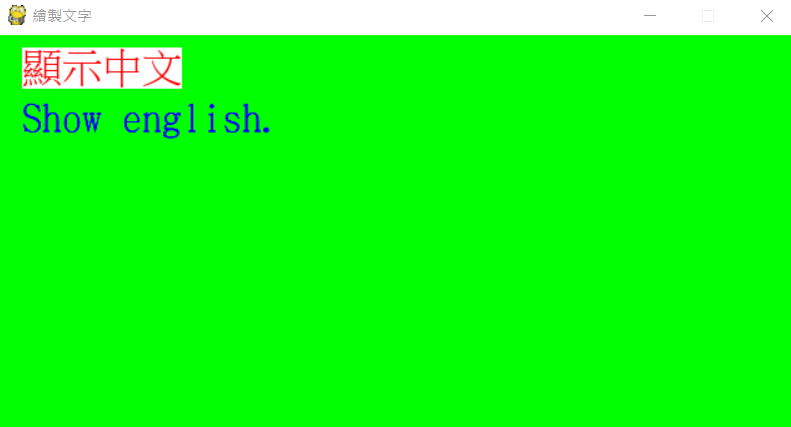# 五、基礎圖形繪製

pygame裡面可以繪制簡單的幾何圖形，

pygame.draw.rect(畫布, 顏色, [x座標, y座標, 寬, 高], 線寬) 矩形
pygame.draw.circle(畫布, 顏色, (x座標, y座標), 線寬) 圓形
pygame.draw.ellipse(畫布, 顏色, [x座標, y座標, x直徑, y直徑], 線寬) 橢圓
pygame.draw.arc(畫布, 顏色, [x座標, y座標, x直徑, y直徑], 起始角(單位為弧度), 結束角, 線寬) 圓弧
pygame.draw.line(畫布, 顏色, (x座標1, y座標1), (x座標2, y座標2), 線寬) 直線
pygame.draw.polygon(畫布, 顏色, 點座標列表, 線寬) 多邊形

``````import pygame
pygame.init()  #pygame初始化

# 設定視窗
width, height = 320, 320
screen = pygame.display.set_mode((width, height))  #建立繪圖視窗
pygame.display.set_caption("繪製基本圖形")  #繪圖視窗標題

# 創建顏色(三個參數為RGB)
GREEN = (0,255,0) # 綠色
RED = (255,0,0) # 紅色
BLUE = (0,0,255) # 藍色
WHITE = (255,255,255) # 白色
BLACK = (0,0,0) # 黑色

# 建立畫布 (書上說通常不會直接在繪圖視窗畫圖)
background = pygame.Surface(screen.get_size())
background = background.convert() #為畫布建立副本，加快顯示速度
background.fill(WHITE)

# 繪製基本圖形
pygame.draw.circle(background, BLACK, (150,150), 130, 4)
pygame.draw.circle(background, BLUE, (100,120), 25, 0)
pygame.draw.circle(background, BLUE, (200,120), 25, 0)
pygame.draw.ellipse(background, GREEN,[135, 130, 30, 80], 0)
pygame.draw.arc(background, RED, [80, 130, 150, 120], 3.4, 6.1, 9)

# 遊戲主要無窮迴圈
running = True
while running:
for event in pygame.event.get():
if event.type == pygame.QUIT:  #使用者按右上角的關閉鈕
running = False
screen.blit(background, (0,0)) #在繪圖視窗繪製畫布
pygame.display.update()  #更新繪圖視窗

pygame.quit()  # 關閉繪圖視窗
``````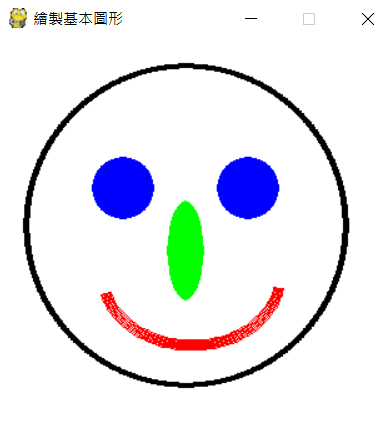# 六、動畫基礎

``````import pygame
pygame.init()  #pygame初始化

# 設定視窗
width, height = 640, 70
screen = pygame.display.set_mode((width, height))  #建立繪圖視窗
pygame.display.set_caption("水平移動")  #繪圖視窗標題

# 創建顏色(三個參數為RGB)
GREEN = (0,255,0) # 綠色
RED = (255,0,0) # 紅色
BLUE = (0,0,255) # 藍色
WHITE = (255,255,255) # 白色
BLACK = (0,0,0) # 黑色
YELLOW = (255,255,0) #黃色

# 建立畫布 (書上說通常不會直接在繪圖視窗畫圖)
background = pygame.Surface(screen.get_size())
background = background.convert() #為畫布建立副本，加快顯示速度
background.fill(WHITE)

# 創建藍色小球(它也有自己的畫布，移動球即等於移動它的畫布)
ball = pygame.Surface((30,30))  #建立球矩形繪圖區
ball.fill((255,255,0))  #這邊我故意將球的背景色設為黃色，以清楚看到球的畫布
pygame.draw.circle(ball, (0,0,255), (15,15), 15, 0)  #畫藍色球
rect1 = ball.get_rect()  #取得球矩形區塊
rect1.center = (320,45)  #球起始中心位置
x, y = rect1.topleft  #球左上角坐標
dx = 3  #球運動速度

# 遊戲主要無窮迴圈
clock = pygame.time.Clock() #重要，計時物件
running = True
while running:
clock.tick(30)  #每秒執行30次
for event in pygame.event.get():
if event.type == pygame.QUIT:  #使用者按右上角的關閉鈕
running = False

# 藍色小球動畫
x += dx  #改變水平位置
rect1.center = (x,y)
if rect1.left <= 0 or rect1.right >= screen.get_width():  #若到達左右邊界，往反向移動
dx *= -1

screen.blit(background, (0,0)) # 在繪圖視窗繪製畫布
screen.blit(ball, rect1.topleft) # 繪製藍色小球
pygame.display.update()  #更新繪圖視窗

pygame.quit()  # 關閉繪圖視窗
``````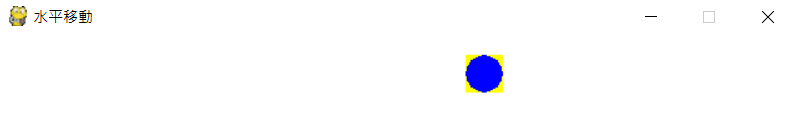<自我學習>

`dx = 3 #球運動速度`
`clock.tick(30) #每秒執行30次`

(試試改`dx = 30``clock.tick(3)`?)

# 參考資料

1. python遊戲編程之旅(共九篇)
2. 書本- Python初學特訓班/ 出版社：碁峰